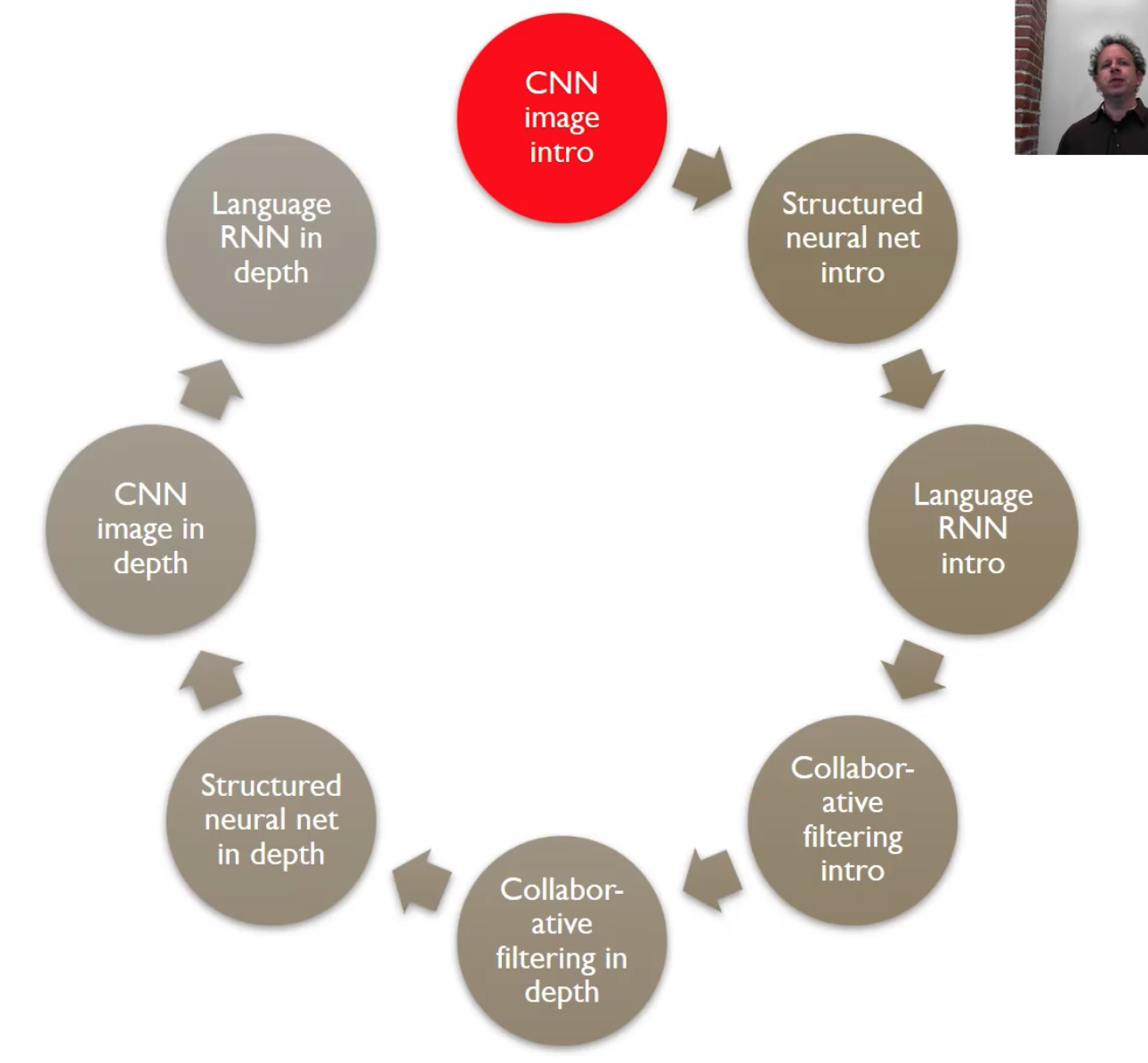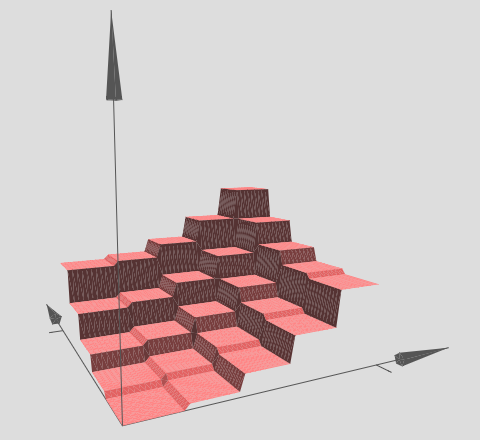# Notes for: Fast.ai 2018 - Lesson 1: Intro to DNN and Image classification

The approach of the class is Top-Down. Get a Neural Network running as fast as possible and understand the concepts later gradually. Thats how you learn sports and music.

The class does assume you know some basic about ML, what a train and valid dataset is, a little bit of numpy and and stuff like that.

Structure of the course:Options to run Jupyter Notebook on public cloud:

## Cats vs Dogs

The images are 198x178x3. It's a 3 dimensional array or a rank 3 tensor.

An epoch is how many times will we look at the whole dataset.

The python fastai library is built on top of pytorch. It wraps a bunch of models such as VGG16 and Resnet50.

After building a model is very helpful to visualize what its build. For example look at the predictions that is very sure its a dog but its actually a cat. Take advantage that is doing great and fix that is doing wrong. This also usually helps to explore big datasets since you will learn something about it.

## Overview

Deep Learning is a kind of Machine Learning

ML used to be very hard, not its relatively easy to get amazing results in cheap hardware. Tasks that are now solved by ML used to require Domain Knowledge and it was very time intensive.

We want to find a function that:

1. Infinitely flexible function: can solve any problem
2. All purpose parameter fitting: If you set the parameters of the function correctly
3. Is fast and scalable.

This function is a Neural Network.

Neural Network consists of a number of linear layers connected to a number of non-linear layers. When you interspace things this way you get an "Universal Approximator", this kind of function can solve any given problem to arbitrary close accuracy as long as you add enough parameters. This is a theorem so its been proven to be an Infinitely flexible function.

Gradient Decent: Is a way to fit the parameters so that the Flexible Function (Neural Network) solves some specify problem. For the parameters I have, how good are they to solve my problem? and figure out a slightly better set of parameters, and iterate until we rich a minimum.

For ML it happens that there is not local minimum there is usually one true solution.

All of this is possible theoretically but we need to do it in a reasonable amount of time. Thats were GPUs come in since they can solve this parameter fitting problem fast and cheap.

A single layer NN, even if it matches the Universal Approximator Theorem, solve any given problem arbitrarily closely, they require an exponentially increasing number of parameters to do it. So its not fast and scalable.

This is solved by adding more hidden layers, this gives us close to linear scalability. So you can add a few more hidden layers to get more accuracy to more complex problems. This is Deep Learning.

At Google from 2012 to 2016 they started to apply DL into a lot of parts of the business because DL can solve any problem.

## Convolutional NN

This is a type of NN that uses convolutions, a linear operation, that transforms an image based on a Kernel (a small 2x2 or 3x3 matrix). The CNN takes each section (could be 2x2 or 3x3) of the image and multiplies it by the Kernel.

Based on the kernel we can find different things about the image. In the demo website if we use the top sobel kernel we can see that its finding the edges of the image. Note that black means nothing (0) while white means an actual value (1) or information.

Since a convolution is a linear operation it can be a layer of a Neural Network

All NN linear layers are followed by a non-linear layer. This non-linearities, take a value and turns it into another value in a non linear way. For example a Sigmoid or a ReLu (`y = max(x, 0)` , more famous now).

If we just did Linear functions, the output will be just one linear function.

The key point of taking a linear layer followed by an element-wise non-linear function is that allows us to create very complex shapes (see: http://neuralnetworksanddeeplearning.com/chap4.html) and this is the key idea on why NN can solve any problem.Gradient decent is based on the derivate of the function generated by the NN. We move into the direction of the derivative an small amount called learning rate, getting this number right is important.

CNN combines all this ideas and extends it to not one kernel but a lot of kernels that are are learned from the data using gradient decent.

This kernels can be visualized (see paper) and multiple layers start go get more information. Layer 1 is edges. Layer 2, combines Layer 1 features, and tells us more shape like objects such as circles, rectangles or horizontal lines. Layer 3, combines Layer 2 features, and it learns to recognize presence of text, faces, petals and more. By Layer 5 can recognize, eyes of birds, bike wheels, dog faces.

The Learning Rate is very important and finding it used to be complicated but there is a some automated ways of doing that now such as see Cyclical Learning Rates for Training Neural Networks that works like this:

1. Start at a random point as usual
2. Take a very tiny step
3. Iterate and increase (double) the learning rate each time, steps are getting bigger and bigger
• So at the beginning it will do almost nothing, then perform very well and after it will start performing poorly
4. Find the best improvement step (just before it became bad), what is the point where it was dropping the fastest and use that Learning Rate. plotting loss vs learning rate is useful for this

If while training loss starts to increase a lot, is likely that the learning rate is to high.

How many epochs (how many times will we look at the whole dataset ) should we do? As many as you can until metrics start to get worst (overfitting).